Courses

# SI And CI Engines - MCQ Test 2

## 30 Questions MCQ Test Mock Test Series - Mechanical Engineering (ME) for GATE 2020 | SI And CI Engines - MCQ Test 2

Description
This mock test of SI And CI Engines - MCQ Test 2 for Mechanical Engineering helps you for every Mechanical Engineering entrance exam. This contains 30 Multiple Choice Questions for Mechanical Engineering SI And CI Engines - MCQ Test 2 (mcq) to study with solutions a complete question bank. The solved questions answers in this SI And CI Engines - MCQ Test 2 quiz give you a good mix of easy questions and tough questions. Mechanical Engineering students definitely take this SI And CI Engines - MCQ Test 2 exercise for a better result in the exam. You can find other SI And CI Engines - MCQ Test 2 extra questions, long questions & short questions for Mechanical Engineering on EduRev as well by searching above.
QUESTION: 1

### A fuel represented by the formula C8H16 is used in an I.E. Engine. Given thatthe molecular weight of air is 29 and that 4.76 kmols of air contain 1 kmol ofoxygen and 3.76 kmol of nitrogen, the Air /Fuel ratio by mass is

Solution:

Ans. (c)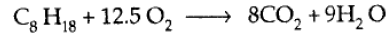QUESTION: 2

Solution:

Ans. (d)

QUESTION: 3

### The stoichiometric air/fuel ratio for petrol is 15: 1.  What is the air/fuel ratio required for maximum power?

Solution:

Ans. (d) For Maximum power Air/Fuel ratio=12:1
For Maximum economy Air/Fuel ratio=16:1
For Maximum power we need rich mixture.

QUESTION: 4

Match List I with List II and select the correct answer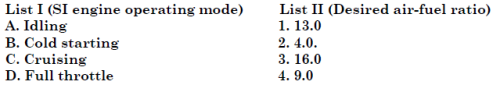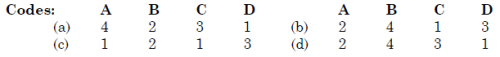Solution:

Ans. (a) Cold starting requires maximum fuel, idling requires little less fuel, full throttle requires still less fuel and idling requires minimum fuel.

QUESTION: 5

Match List I (Air-fuel ratio by mass) with List II (Engine operation mode) and select the correct answer using the codes given below the Lists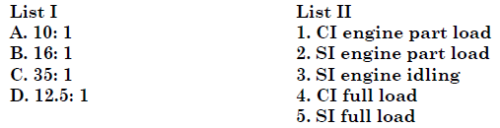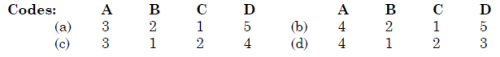Solution:

Ans. (a)

QUESTION: 6

Auto -ignition time for petrol- air mixture is minimum when the ratio of actual fuel-air ratio and chemically correct fuel air ratio is

Solution:

Ans. (c)

QUESTION: 7

Assertion (A): In SI engines, as the engine speed increases, spark is required to be advanced.Reason (R): As the engine speed increases, flame velocity increases.

Solution:

Ans. (c)

QUESTION: 8

By higher octane number of SI fuel, it is meant that the fuel has

Solution:

Ans. (d) A fuel with higher octane number has longer ignition delay.

QUESTION: 9

Consider the following statements:
1. In the SI engines detonation occurs near the end of combustion whereas in CI engines knocking occurs near the beginning of combustion.
2. In SI engines no problems are encountered on account of pre-ignition.
3. Low inlet pressure and temperature reduce knocking tendency in SI engines but increase the knocking tendency in CI engines.
Which of the statements given above are correct?

Solution:

Ans. (d)

QUESTION: 10

List I gives the different terms related to combustion while List II gives the outcome of the events that follow. Match List I with List II and select the correct answer.
List I                                           List II
A. Association                           1. Pseudo shock
B. Dissociation                          2. Knock
C. Flame front                           3. Endothermic
D. Abnormal combustion        4. Exothermic
Code: A B C D                     A B C D
(a) 3 4 1 2                (b) 4 3 1 2
(c) 3 4 2 1                (d) 4 3 2 1

Solution:

Ans. (b)

QUESTION: 11

Consider the following statements:
In order to prevent detonation in a spark-ignition engine, the charge awayfrom the spark plug should have
1. Low temperature
2. Low density
3. Long ignition delay
Which of the statements given above is/are correct?

Solution:

Ans. (d)

QUESTION: 12

Which of the following action(s) increaser(s) the knocking tendency in the S I.engine?

Solution:

Ans. (c)

QUESTION: 13

In a SI engine, combustion stage I takes 1 ms and combustion stage II takes1·5 ms when the engine runs at 1000 rpm. If stage I time duration isindependent of engine speed what will be the additional spark advancenecessary when the engine speed is doubled?

Solution:

Ans. (b)

QUESTION: 14

Consider the following statements:
Knock in the SI engine can be reduced by
1. Supercharging
2. Retarding the spark.
3. Using a fuel of long straight chain structure
4. Increasing the engine speed.
Of these correct statements are

Solution:

Ans. (d) Knock in SI engine can be reduced by retarding spark and increasing the engine speed.

QUESTION: 15

Consider the following statements:
Detonation in the S.I. engine can be suppressed by
1. Retarding the spark timing.
2. Increasing the engine speed.
3. Using 10% rich mixture.
Of these statements

Solution:

Ans. (d) Decreasing the engine speed increases possibility of detonation

QUESTION: 16

Consider the following statements relevant to the ignition system of SI engine:
1. Too small a dwell angle will lead to the burning of condenser and contactpoints
2. Too small a dwell angle will result in misfiring.
3. Too large a dwell angle will result in burning of condenser and contactpoints.
4. Too large a dwell angle will result in misfiring.
Which of the above statements are correct?

Solution:

Ans. (b)

QUESTION: 17

Which of the following feature(s) is/are used in the combustion chamber design to reduce S.I engine knock?

Solution:

Ans. (c)

QUESTION: 18

A two-stroke engine has a speed of 750 rpm. A four-stroke engine having anidentical cylinder size runs at 1500 rpm. The theoretical output of the two stroke engine will

Solution:

Ans. (c) In two-stroke engine there is one power stroke in 2 strokes, but in four-stroke
engine there is one power stroke in 4 strokes.

QUESTION: 19

If the approximate average mean pressures during induction, compression,power and exhaust strokes of an internal combustion engine are respectively15 kN/m2 below atmosphere, 200 kN/m2 above atmosphere, 1000 kN/m2 above atmosphere and 20 kN/m2 above atmosphere, then the resultant mean effective pressure, in kN/m2 , is

Solution:

Ans. (a) Resultant mean effective pressure = 1000 - 200 - (15 + 20) = 800 - 35 = 765 kN/m2

QUESTION: 20

Which of the following statements is "true"?

Solution:

Ans. (a)

QUESTION: 21

Assertion (A): A very high compression ratio is favoured for a CI engine, in order toattain high mechanical efficiency without knocking.
Reason (R): The delay period in CI combustion affects rate of pressure rise and hence knocking.

Solution:

Ans. (d) A very high compression ratio is favoured for a CI engine, in order to attain high THERMAL efficiency without knocking.

QUESTION: 22

Consider the following measures:
1. Increasing the compression ratio
2. Increasing the intake air temperature.
3. Increasing the length to diameter ratio of the cylinder.
4. Increasing the engine speed.The measures necessary to reduce the tendency to knock in CI engines wouldinclude

Solution:

Ans. (a)

QUESTION: 23

The knocking tendency in compression ignition engines increases with:

Solution:

Ans. (c)

QUESTION: 24

Which of the following factors would increase the probability of knock in theCI engines?
1. Long ignition delay of fuel
2. High self ignition temperature of fuel
3. Low volatility of fuel
Select the correct answer using the codes given below:

Solution:

Ans. (a)

QUESTION: 25

Hypothetical pressure diagram fora compression ignition engine is shown in the given figure. The diesel knock is generated during the period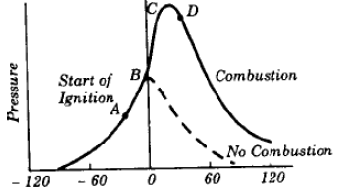Solution:

Ans. (b)

QUESTION: 26

Which of the following factor(s) increase(s) the tendency for knocking in theC.I. engine?

Solution:

Ans. (b) Increasing coolant temperature will reduce knocking in C I engine.

QUESTION: 27

The object of providing masked inlet valve in the air passage of compressionignitionengines is to

Solution:

Ans. (a)

QUESTION: 28

The tendency of knocking in C I engine reduces by

Solution:

Ans. (c)

QUESTION: 29

If the performance of diesel engines of different sizes, cylinder dimensionsand power ratings are to be compared, which of the following parameters canbe used for such comparison?

Solution:

Ans. (c)

QUESTION: 30

In the operation of four-stroke diesel engines, the term ‘squish’ refers to the:

Solution:

Ans. (c) Mixing of the fuel more thoroughly. Improved mixing had to be accomplished by imparting additional motion to the air, most commonly by induction-produced air swirls or a radial movement of the air, called squish, or both, from the outer edge of the piston toward the centre. Various methods have been employed to create this swirl and squish.